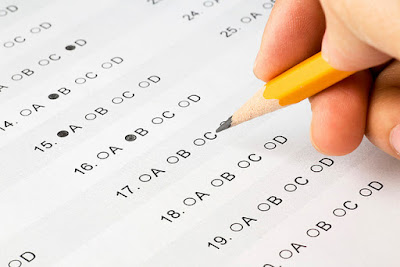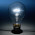## Sunday, November 19, 2017

### A Guide to Negative Marking

Negative marking in Multiple Choice Answer(MCQ) exams is becoming more mainstream in competitive exams all across India. The intent of negative marking is to prevent students from taking guesses at answers. It is typically quite effective because of loss aversion.CC License
Before we move onto the analysis. Some definitions:

 Term Definition NumChoices Number of Choices of answers in an MCQ NegRatio The ratio of the number of positive marks one gets for a correct answer to the number of negative marks one gets for an incorrect answer. If it is a +4 and -1 exam, the ratio is 4. If it is a +1 and -2 exam, the ratio is 0.5 Random Strategy Strategy to mark the answers not known, at random Refrain Strategy Strategy to refrain from guessing, if the answer is unknown

## Analysis Level 1:

On the top level, the probabilities seems simple. For example:

If there is an exam with the NumChoices as 4 and NegRatio as 4, the the Math works like this:

 Probability of getting answer correct (Pc) = 0.25 Probability of getting answer incorrect (Pi) = 0.75 Marks probabilistically obtained = (Pc * correct answer marks) + ( Pi * incorrect answer marks) = (0.25 *4) + (0.75*-1) = 0.25

In fact, for different NumChoices and Neg Ratio this table can be used to predict marks obtained by Random Strategy at average, given large number of attempts.

 NegRatio -> 0.5 1 2 3 4 5 NumChoice 2 -0.25 0 0.5 1 1.5 2.00 3 -0.50 -0.33 0 0.33 0.67 1 4 -0.63 -0.5 -0.25 0 0.25 0.5 5 -0.70 -0.6 -0.4 -0.2 0 0.2 6 -0.75 -0.67 -0.5 -0.33 -0.17 0

This analysis however does not tell one too much about what is the right strategy, because it doesn’t tell you how often does the guessing strategy fail you. To better understand we need the Lottery Analogy.

#### Lottery Analogy

Imagine there is a lottery with a 1 in a billion chance to win ₹6 billion. The lottery ticket costs ₹5. By the probability analogy just established, the lottery ticket is worth ₹6 billion / 1 billion = ₹6. So therefore by above practice, it makes sense to always buy the ticket. But for a poor man, whose livelihood depends on the ₹5, it would be stupid to invest in the lottery because there is 99.999999% chance he will starve.

Similarly, if you are in a competitive exam with only one shot, how do you as a student decide, if this one shot is worth the risk. Or what is a probability of getting a zero or greater score by the random strategy?

For the analysis so far - only in the unique situation where the probabilistic result is zero, is there clarity over there being 50% chance of the Random Strategy being better than the Refrain Strategy.

## Analysis Level 2:

This time we will have to move away from direct probability and move to a more brute force solution. For starters, we will simulate someone taking the tests with 100 questions being answered at random from 4 choices with +4 for a correct answer and -1 for an incorrect answer 1,00,000 times.

The scores from the simulation look like this -

The above plot indicates the % of tests in the simulation in which the score was higher than the corresponding number indicated on horizontal axis.

So, interestingly for this case only 10% of the time does one end up with a net negative score on randomly guessed questions using the Random strategy. On the other hand there is the same 10% chance to get 57 free marks. The average as predicted by the table above is around 25 marks. So Random Strategy could be very beneficial.

Here is the same plot for +3 and  -1 type of exam. The gaps between the columns are because in this scenario there are some score that are not possible.

As expected from the table above there is close to 50% chance of the Random Strategy doing better than the Refrain Strategy.

This gets a little bit worse for +2 and -1

## Analysis Level 3:

One is not always guessing

There is the possibility of eliminating at least one answer. For the +4 and -1 case the plot shifts to this

Significantly, not only has the average moved to 70, but also the chance of random strategy failing you is now down to 0.1% or 1 in a thousand.

On the test side, there is also the chance that the exam does not have randomised options. That is the options A, B, C and D are in the order the question setter wrote them. Typically, a test has options like "All of the above" or "None of the above" are not randomised. In these situations, the probability of the following option being the correct answer is this:

A B C D
20% 40% 30% 10%

If the simulations are run with this in mind and the answer entered is always B, then the distribution for the Random Strategy looks like this-

Now no test in the simulation scored < 0. In fact the average positive gain has moves to 127 marks.

Finally, typically there is a hunch that one might have, let's say that means that the probability of the hunch being correct is 50%. In that situation the distribution shift looks like this -

No test in the simulations with score < 0 and Average moves upto 152.

## Conclusion:

For negative marking exams with NumChoice = 4 and NegRatio > 3, Random Strategy is looking strong. Specifically, in situations where the test is non-randomised, an option is eliminated or there is a strong hunch, there is almost no possible way to be negatively affected by the Random Strategy.

1.Man! Where were you 15 yrs ago! :)

1.Wait, you were giving Negative marks exam 15 years ago?

2.I am really pleased to post my comment on this blog .I love your blog by the way, I am gonna have to add you to my list of watched blogs. Very Nice blog post. I found this article very informative & useful. Keep sharing. The given information is really very helpful. Thanks a lot for providing such a useful information. There is a wide range of online casinos games to choose from, and all of them offer different payouts, prizes and even bonus features. Chiêm bao thấy đi chơi với người yêu cũ

3.What a fantabulous post this has been. Never seen this kind of useful post. I am grateful to you and expect more number of posts like these. Thank you very much. The formula will predict the best numbers and then suggests the best way to combine them together giving the punter a greater chance of winning a big prize or have several or multiple winning lines. mơ thấy bạn trai cũ đánh con gì

4.